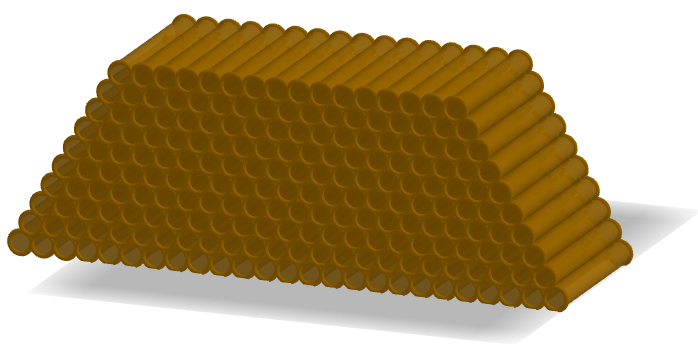# How to make a 3D trapezoidal pile with cylinders?

jospercomp shared this question 2 years ago

How to make a 3D trapezoidal pile with cylinders and two sliders?

a slider with the number of cylinders in the first row

and a slider with the number of layers.l1=Flatten(Sequence(Sequence((2r k - r j, (-h) / 2, r + sqrt(3) r (n - 1 - j)), k, 0, j), j, n - l, n - 1))

l2=Flatten(Sequence(Sequence((2r k - r j, h / 2, r + sqrt(3) r (n - 1 - j)), k, 0, j), j, n - l, n - 1))

Zip(Cylinder(P1, P2, 1), P1, l1, P2, l2)1

l1=Flatten(Sequence(Sequence(Sequence((2r k - r j, (-h) / 2, r + 2 sqrt(2) / sqrt(3) r (n - 1 - j)), m, 0, l), k, 0, j), j, 0, n - 1))

l2=Flatten(Sequence(Sequence(Sequence((2r k - r j, h / 2, r + 2 sqrt(2) / sqrt(3) r (n - 1 - j)), m, 0, l), k, 0, j), j, 0, n - 1))

Zip(Cylinder(P1, P2, 1), P1, l1, P2, l2)

I would need to restrict the number of levels or layers.1

l1=Flatten(Sequence(Sequence((2r k - r j, (-h) / 2, r + 2sqrt(2) / sqrt(3) r (n - 1 - j)), k, 0, j), j, n - l, n - 1))

l2=Flatten(Sequence(Sequence((2r k - r j, h / 2, r + 2sqrt(2) / sqrt(3) r (n - 1 - j)), k, 0, j), j, n - l, n - 1))1

Your z-coordinate is off again, the cylinders overlap. Replace the "2sqrt(2) / sqrt(3)" by "sqrt(3)". (It's just based on the pythagorean theorem in a midpoint triangle.)

Also, in your "cylinder(P1, P2, 1)", you should probably use radius r instead of 1. Or if you want fixed radius r=1, then you can get rid of the r in your l1, l2.1

Thank you!!!1

l1=Flatten(Sequence(Sequence((2r k - r j, (-h) / 2, r + sqrt(3) r (n - 1 - j)), k, 0, j), j, n - l, n - 1))

l2=Flatten(Sequence(Sequence((2r k - r j, h / 2, r + sqrt(3) r (n - 1 - j)), k, 0, j), j, n - l, n - 1))

Zip(Cylinder(P1, P2, 1), P1, l1, P2, l2)2

así

Files: foro.ggb